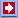Software Online Library ContactsSoftware: Business&GamesCalculator Download Analyzer Mail NavigatorOnline: Stat Calc Y(X) Finder 2D Plotter 3D Plotter Science CalcLibrary: Data Mining Statistics Machine Learning Genetic Algorithms Neuronets Fuzzy logic ForecastLinksContacts## Tools

• QuData Statistical Calculator - allows to calculate different statistical parameters of the sample of random numbers.
• QuData Y(X) Finder - Allows to fit empirical data (xi, yi) with the most suitable analytical function y=y(x).
• 2D Plotter - Allows to plot graphs of three functions and one set of empirical data.
• 3D Plotter - Builds the surface plot of a function that depends on two variables.
• Sci Calc - Scientific calculator with a lot of functions.